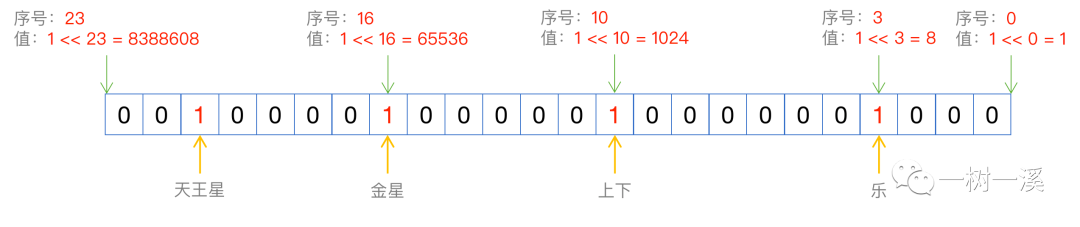# MySQL 简单查询语句执行过程分析（四）WHERE 条件

chujichenxuyuan

0收藏

where 条件结构

where 条件比较

• set 字段
• enum 字段
• bit 字段

``````CREATE TABLE `t_recbuf` (
`id` int(10) unsigned NOT NULL AUTO_INCREMENT,
`i1` int(10) unsigned DEFAULT '0',
`str1` varchar(32) DEFAULT '',
`str2` varchar(255) DEFAULT '',
`c1` char(11) DEFAULT '',
`e1` enum('北京','上海','广州','深圳','天津','杭州','成都','重庆','苏州','南京','洽尔滨','沈阳','长春','厦门','福州','南昌','泉州','德清','长沙','武汉') DEFAULT '北京',
`s1` set('吃','喝','玩','乐','衣','食','住','行','前后','左右','上下','里外','远近','长短','黑白','水星','金星','地球','火星','木星','土星','天王星','海王星','冥王星') DEFAULT '',
`bit1` bit(8) DEFAULT b'0',
`bit2` bit(17) DEFAULT b'0',
`blob1` blob,
`d1` decimal(10,2) DEFAULT NULL,
PRIMARY KEY (`id`)
) ENGINE=InnoDB AUTO_INCREMENT=2001 DEFAULT CHARSET=utf8;``````

1. where 条件结构

MySQL 中多层 where 条件会形成一棵树状结构，每多一个层级，都需要额外的逻辑处理，执行效率上会有一点影响，所以在语法分析阶段，就会对 where 条件的树状结构层级进行简化，可以合并的层级就合并。

``````select * from t_recbuf
where (i1 > 1024 and e1 = '成都')
or (d1 > 1020.05 and d1 < 1048576.88)``````

Item_func_gt 类实例，表示 i1 > 1024

Item_func_eq 类实例，表示 e1 = '成都'

• Item_func_gt 类实例，表示 d1 > 1020.05
• Item_func_lt 类实例，表示 d1 < 1048576.88

2. where 条件比较

``````// 以下代码中，各行代码之间也省略了其它无关的代码
Item *condition= qep_tab->condition();
bool found= TRUE;

if (condition)
{
found= MY_TEST(condition->val_int());
}``````

• 如果为 false，结束循环，Item_cond_and 条件最终结果为 false。
• 如果为 true，还要判断接下来的 Item_func_eq 条件(e1 = '成都')。

• 如果为 true，则 Item_cond_and 条件最终结果为 true。
• 如果为 false，则 Item_cond_and 条件最终结果为 false。

Item_func_eq 条件(e1 = '成都' )中有一个属性 func，是用来比较存储引擎返回的 e1 字段的值是否等于成都的，func 属性在我们讲第二篇(查询准备阶段)时提到过，func 属性的值就是在查询准备阶段确定的，对于 e1 = '成都' 这个 where 条件，func 设置为字符串比较函数。

3. 三种特殊类型字段怎么比较?

3.1 set 字段

set 类型的字段在 InnoDB 中以整数存储，字段返回给 server 层时也是整数，定义表结构时指定的每一个选项占用 1 bit。

• 乐是第 4 个选项，序号为 3，值为 1 << 3 = 8。
• 上下是第 11 个选项，序号为 10，值为 1 << 10 = 1024。
• 金星是第 17 个选项，序号为 16，值为 1 << 16 = 65536。
• 天王星是第 22 个选项，序号为 21，值为 1 << 21 = 2097152。

s1 字段的整数值由 4 个选项按位或计算得到：8 | 1024 | 65536 | 2097152 = 2163720，二进制示意图如下：server 层读取到 s1 字段的整数值之后，遍历 s1 字段的 24 个选项，判断整数值中选项对应的 bit 是否为 1，如果为 1，则把该选项文本(如上下)追加到 s1 字符串值的后面，用逗号分隔。

``````select * from t_recbuf
where s1 = '水星,金星'``````

``````select * from t_recbuf
where find_in_set('金星', s1)``````

3.2 enum 字段

``````select * from t_recbuf
where e1 = '成都'``````

``````select * from t_recbuf
where e1 = 7``````

3.3 bit 字段

bit 类型的字段，存储引擎以 C/C++ 中的 char 指针指向一块内存区域的形式，把字段内容返回给 server 层，server 层会把 char 指针指向的内存区域的内容转换为 where 条件中的值类型，然后进行比较。

``````select * from t_recbuf
where bit1 = 220``````

``````select * from t_recbuf
where bit1 = b'11011100'``````

``````select * from t_recbuf
where bit1 = bit1 | b'100'``````

``````-- 把 b'100' 替换为 4，效果是一样的
select * from t_recbuf
where bit1 = bit1 | 4``````帖子
视频
声望
粉丝
最近发布
社区精华内容